# ROLL-UP

This operator was proposed in Gray et al. (1996), and subsequently in Cabibbo & Torlone (1997), in Gray et al. (1997), in Lehner (1998), in Nguyen, Tjoa, & Wagner (2000), and in Pedersen, Jensen, & Dyreson (2001). A roll-up on a dimension hierarchy of a MAD means giving "less detail" by ascending this dimension hierarchy, aggregating with an implicit aggregation function. This corresponds to performing aggregate formation on "higher" category types with the given aggregate function. Sometimes, we also need a reference to the original MAD in this case. This is caused by the possible non-summarizability in the MAD, which means that we cannot necessarily combine the aggregate results from intermediate levels into higher-level results, but we need to compute the result directly from the lowest-level data (base data).

Reports commonly aggregate data at a coarse level, and then at successively finer levels. The car sales report in Figure 18 shows the idea. Data is aggregated by model, then by year, then by city. If the measure is summable and the MAD is well formed, as defined in Chapter 4, and if, obviously, another level at a higher level exists along the hierarchy, then the roll-up operator is applicable and the end result is a new MAD with more aggregate measure instances.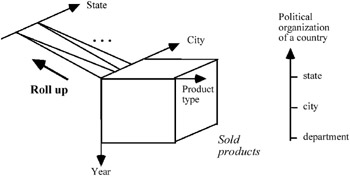Figure 18

The roll-up carried out on the category attribute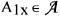of a given MAD s1 produces a new MAD s'1 defined on the same descriptive space schema, but with the category attribute Ex, on which the roll-up was applied, substituted by the category attribute E'x of the higher level of the hierarchy defined in the relative dimension, i.e.: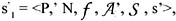where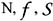are the same and P' is the new name of the MAD;

s' is a set of recomputed summary values. This recomputation depends on the type of aggregation function (e.g., count, sum, average) applied to the original microdata.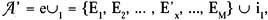where the hierarchy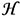of the dimension which the roll-up was applied to is Ex E'x,.

In Gray et al. (1996), and subsequently in Gyssens & Lakshamanan (1997), the authors propose a refinement of the above-mentioned operator, namely the monotone roll-up operator.

In many applications, in fact, only certain fragments of the MAD are of interest. In general, if X is the set of descriptive variables of the MAD and Y is a subset of X, then only aggregates regarding Y and all its subsets may be of interest.Multidimensional Databases: Problems and Solutions
ISBN: 1591400538
EAN: 2147483647
Year: 2003
Pages: 150